## Validating ZIP Codes with CDXZipStream

A simple but important application of CDXZipStream is testing zip code validity. Particularly when dealing with large address lists, CDXZipStream can quickly and easily eliminate the inconvenience and cost associated with invalid or improperly entered zip codes. Included here are examples of how CDXZipStream answers the question: Are your zip codes real?

First, we recommend that when obtaining zip code data from CDXZipStream that you request the zip code value be returned as well - this will provide confirmation of how CDXZipStream is interpreting the original zip code input, as shown below: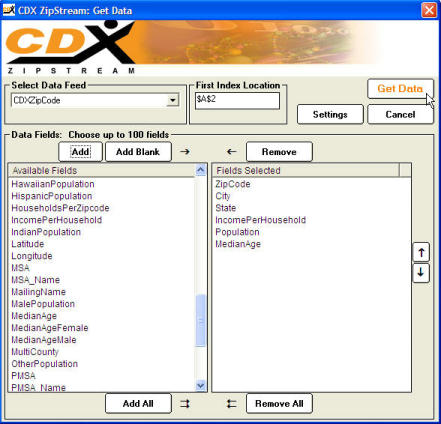The result from the data request above is: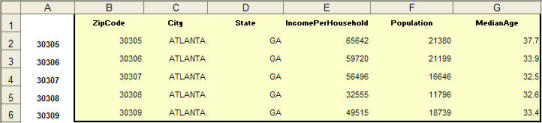(Note - the data returned from CDXZipStream are highlighted in yellow in columns B through G. Original zip code input is in column A.)

All the original zip codes in the case above appear valid. The zip codes returned by CDXZipStream are exactly the same as the original input zip codes in Column A. No problems here. But what happens in the following cases?

Case 1: Zip Code does not exist

If the zip code does not exist, due to a data entry problem or some other error, the returned values from CDXZipStream will return "Zip Code Not Found":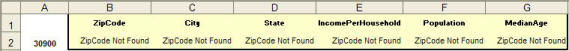If the original input zip code is entered in a cell that that has general or number formatting, Excel will interpret the zip code to be a number value and will drop any leading zeroes. For example, the zip code 08033 will display as the number 8033. In this case CDXZipStream will automatically add a leading zero to any four digit input, as shown below: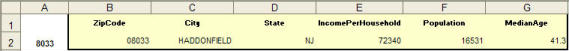You may want to consider formatting any cells containing input zip codes as text to retain leading zeros and avoid confusion.

Case 3: Extended Zip Code + 4 input

CDXZipStream will automatically truncate an extended zip code in order to ensure data will be returned for the 5-digit code. This is true even in cases where the leading zero is dropped (in cell A3), and even in cases where the 4 digit extension is incorrectly entered (in cell A4):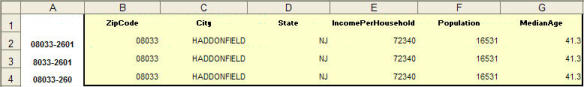Case 4: Zip Code exists but is invalid for the input address

This is a common situation where the zip code exists but is not valid for the input address. In this case the input city and state can be checked against returned city and state data from CDXZipStream.

In row 2 below, the input zip code 08043 exists but is invalid, since the input city and state (Cherry Hill, NJ) does not match the city and state returned by CDXZipStream (Voorhees, NJ). Row 3 shows the correct zip code, with matching city and state combinations.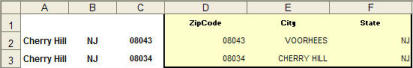Checking for Discrepancies

For large address lists, we recommend that you use a logical formula to detect when there are discrepancies in the data. This allows you to quickly and easily identify problem areas. For Case 4 above, we can use the following formula in cell G2 to test for city and state matches:

=IF (OR (A2<> E2, B2<>F2), 1,"")

If either city or state data do not match, the formula will place the number 1 in cell G2. If city and state both match, the cell will be empty. This formula can then be copied to a longer list of data: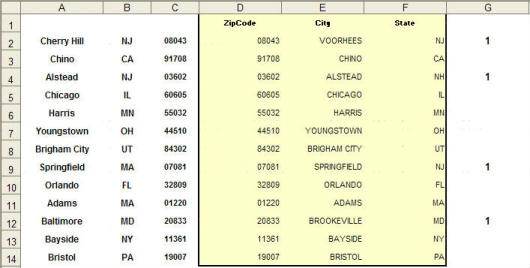Using a logical formula in column G, four discrepancies in city or state data were identified. This method can also be used to check zip code discrepancies as well. For the case above (in Row 2):

=IF (LEFT(C2,5)<> LEFT(D2,5), 1,"")

This will alert you when a zip code does not exist or when a leading zero has been dropped from the input zip code. Note that the LEFT function in this formula will truncate the last four digits in an extended zip code.